### Probability Class 10th Mathematics Gujarat Board Solution

##### Question 1.15 defective ballpens are accidentally mixed with 135 good ones. It is not possible to just look at a ballpen and say whether it is defective or not. One ballpen is picked up at random from it. Find the probability that the ballpen selected is a good one.Answer:Given, Total number of defective ballpens = 15and total number of good ballpens = 135Therefore, total number of ball pens = number of defective ballpens + number of good ball pensSo, total number of ball pens = 15 + 135 = 150Now, let E be the event that the ballpen picked is defective and F be the event that the ballpen picked is good.Therefore, the number of favourable outcomes of event E = 15and , the number of favourable outcomes of event F = 135Total number of favourable outcomes = 15 + 135 = 150Probability that the ball pen selected is good = Probability of event F occuring = P(F)P(F) =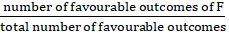=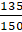= 0.9So, the probability that the ballpen selected is good is 0.9.Question 2.A box contains 5 green, 8 yellow and 7 brown balls. One ball is taken out from a box at random. What is the probability that the ball taken out is (i) yellow ? (ii) brown? (ii) neither green nor brown ? (iv) not brown?Answer:Number of green balls in the box = 5Number of yellow balls in the box = 8Number of brown balls in the box = 7Total number of balls including green, yellow and brown in the box = 5 + 8 + 7 = 20Now, let the event that a green ball is taken from the box be G.the event that a yellow ball is taken from the box be Y.the event that a green ball is taken from the box be B.Number of favourable outcomes of event G = 5Number of favourable outcomes of event Y = 8Number of favourable outcomes of event B = 7Total number of favourable outcomes = 5 + 8 + 7= 20(i) Probability of a yellow ball to be taken from the box = Probability of event Y occurring = P(Y)P(Y) =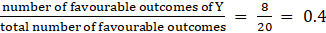(ii) Probability of a brown ball to be taken from the box = Probability of event B occurring = P(B)P(B) =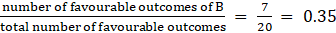(iii) Probability of the taken ball is nether green nor brown = 1 – Probability of ball taken is either green or brown = 1- P(G or B)P(G or B)’ = 1-P(G or B)=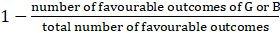=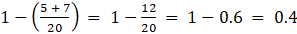(iv) Probability of the ball taken is not brown = 1-Probability of the ball taken is brown = 1-P(B)P(B’) = 1- P(B)=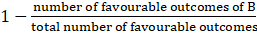=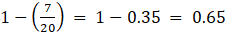Question 3.A bag contains orange flavoured candies only. Rahi takes out one candy without looking into the bag. What is the probability that she takes out (i) the orange flavoured candy? (ii) a lemon flavoured candy?Answer:Let the total number of orange candies in the bag be T.As the bags contain only orange flavoured candies so the total number of candies in the bag= TLet the event that an orange candy is taken out be O.Number of favourable outcomes of the event O = TAlso, total number of favourable outcomes = T(i) Probability that the orange flavoured candy is taken out = Probability of event occurring O = P(O)P(O) =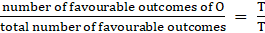= 1(ii) Probability that a lemon flavoured candy is taken out = Probability that an orange flavoured candy is not taken out = P(O’)P(O’) = 1 – P(O) = 1 – 1 = 0Question 4.A box contain 100 cards marked with numbers 1 to 100. If one card is drawn from the box, find the probability that it bears (i) single digit number, (ii) two-digit numbers (iii) three-digit number (iv) a number divisible by 8 (v) a multiple of 9, (vi) a multiple of 5.Answer:Given, total number of cards in the box is 100 with each card marked with numbers from 1 to 100.Therefore, we know that from 1 to 100 , number of single digit numbers = 1 to 9 = 9Also, number of two-digit numbers from 1 to 100 = 10 to 99 = 90Only three digit number from 1 to 100 is 100.So, number of three-digit numbers from 1 to 100 = 1Numbers divisible by 8 from 1 to 100 are 8,16,24,32,40,48,56,64,72,80,88,96 = 12Numbers which are multiple of 9 are 9,18,27,36,45,54,63,72,81,90,99 = 11Numbers which are multiple of 5 are 5,10,15,20,……….100 = 20Now, let the event that a single digit number is drawn from the box be A.Event that card bearing a two-digit number is drawn from the box be B.Event that a card bearing a three-digit number is drawn from the box be C.Event that a card bearing a number divisible by 8 is drawn from the box be D.Event that a card bearing a number which is multiple of 9 is drawn from the box be E.Event that a card bearing a number which is multiple of 5 is drawn from the box be FNumber of favourable outcomes of event A = 9Number of favourable outcomes of event B = 90Number of favourable outcomes of event C = 1Number of favourable outcomes of event D = 12Number of favourable outcomes of event E = 11Number of favourable outcomes of event F = 20Total number of favourable outcomes = 100(i) Probability of the card bearing a single digit number = Probability of occurrence of event A = P(A)P(A) =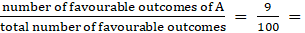0.09(ii) Probability of the card bearing a two-digit number = Probability of occurrence of event B = P(B)P(B) =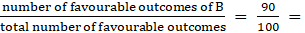0.9(iii) Probability of the card bearing a three-digit number = Probability of occurrence of event C = P(C)P(C) =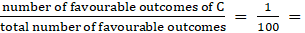0.01(iv) Probability of the card bearing a number divisible by 8 = Probability of occurrence of event D = P(D)P(D) =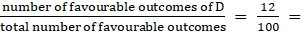0.12(v) Probability of the card bearing a number which is multiple 9 = Probability of occurrence of event E = P(E)P(E) =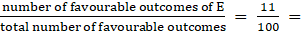0.11(vi) Probability of the card bearing a number which is multiple 5 = Probability of occurrence of event F = P(F)P(F) =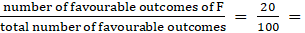0.2Question 5.A carton consist of 100 trousers of which 73 are good, 12 have minor defects and 15 have major defects. Kanu, a trader, will only accept the trousers which are good, but Radha, another trader, will only reject the trousers which have major defects. One trouser is drawn at random from the carton. What is the probability that, (i) it is acceptable to Kanu ? (ii) it is acceptable to Radha?Answer:Total number of trousers = 100Number of trousers which are good = 73Number of trousers with minor defects = 12Number of trousers with major defects = 15Now, let the event that a trouser is acceptable to Kanu be E.Event that a trouser is acceptable to Radha be F.Number of favourable outcomes of event E = 73Number of favourable outcomes of event F = 15Total number of favourable outcomes = 100(i)Probability that a trouser is acceptable = Probability of occurrence of event E = P(E)P(E) =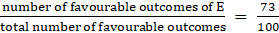= 0.73(ii)Probability that a trouser is not acceptable = Probability of occurrence of event F = P(F)P(F) =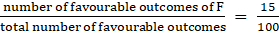= 0.15Question 6.Marks obtained by 50 students from 100 are as follows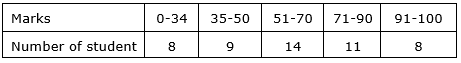Find the probability that a student get marks:(i) below 34, (ii) between 71-90(iii) more than 70(iv) less than or equal to (v) above 90.Answer:The above chart shows the marks obtained by 50 students from total marks 100.Total marks = 100Total number of students = 50Number of students scoring between 0-34 = 8Number of students scoring between 35-50 = 9Number of students scoring between 51-70 = 14Number of students scoring between 71-90 = 11Number of students scoring between 91-100 = 8Now, Let the event that students getting marks below 34 be A.Event that students getting marks between 71-90 be B.Event that students getting marks more than 70 be C.Event that students getting marks less than or equal to 50 be D.Event that students getting marks above 90 be E.Number of favourable outcomes of event A = 8Number of favourable outcomes of event B = 11Number of favourable outcomes of event C = 11 + 8 = 19Number of favourable outcomes of event D = 8 + 9 = 17Number of favourable outcomes of event E = 8(i) Probability that a student get marks below 34 = Probability of occurrence of event A = P(A)P(A) =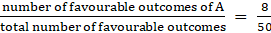= 0.16(ii) Probability that a student get marks between 71-90 = Probability of occurrence of event B = P(B)P(B) =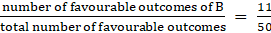= 0.22(iii) Probability that a student get marks more than 70 = Probability of occurrence of event C = P(C)P(C) =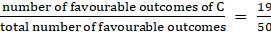= 0.38(iv) Probability that a student get marks less than or equal to 50 = Probability of occurrence of event D = P(D)P(D) =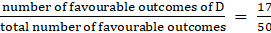= 0.34(v) Probability that a student get marks above 90 = Probability of occurrence of event E = P(E)P(E) =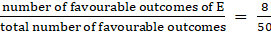= 0.16Question 7.Two fair dice are rolled simultaneously. Find the probability of the following events:(1) A : getting the same number on both dice. (2) B : the sum of the integers on two dice is more than 4 but less than 8.(3) C : the product of numbers on two dice is divisible by 2.(4) D : the sum of numbers on two dice is greater than 12.Answer:If two dice are rolled together then we get 36 outcomes as(1,1),(1,2),(1,3),(1,4),(1,5),(1,6)(2,1),(2,2) ,(2,3),(2,4),(2,5) (2,6)(3,1),(3,2) ,(3,3),(3,4),(3,5) (3,6)(4,4),(4,2) ,(4,3),(4,4),(4,5) (4,6)(5,5),(5,2),(5,3),(5,4),(5,5) (5,6)(6,1),(6,2) ,(6,3),(6,4),(6,5) (6,6)Favourable outcomes of event A i.e, getting the same number on both sides are (1,1), (2,2), (3,3), (4,4), (5,5), (6,6)Number of favourable outcomes of event A = 6Favourable outcomes of event B i.e, the sum of the integers on two dice is more than 4 but less than 8 are (1,4), (1,5), (1,6), (2,3), (2,4),(2,5), (3,2),(3,3), (3,4), (4,1), (4,2), (4,3), (5,1), (5,2), (6,1),Number of favourable outcomes of event B = 15Favourable outcomes of event C i.e, the product of numbers on two dice is divisible by 2 are(1,2),(1,4),(1,6)(2,1),(2,2) ,(2,3),(2,4), (2,5)(2,6)(3,2),(3,4)(3,6)(4,1),(4,2) ,(4,3),(4,4) ,(4,5),(4,6)(5,2),(5,4)(5,6)(6,1),(6,2) ,(6,3),(6,4),(6,5),(6,6)Number of favourable outcomes of event C = 27Favourable outcomes of event D i.e, the sum of numbers on two dice is greater than 12 is 0 as the greatest value of the sum of numbers on two dice is 6 + 6 = 12.Number of favourable outcomes of event D = 0Total number of favourable outcomes = 36(i) Probability of occurrence of event A = P(A)P(A) =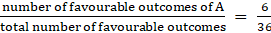= 0.16667(ii) Probability of occurrence of event B = P(B)P(B) =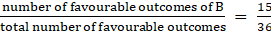= 0.41667(iii) Probability of occurrence of event C = P(C)P(C) =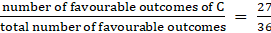= 0.75(iv) Probability of occurrence of event D = P(D)P(D) =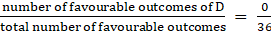= 0Question 8.A coin is tossed three times. Find the probability of the following events:(1) A: getting at least two heads,(2) B: getting exactly two heads,(3) C: getting at most one head,(4) D: getting more heads than tails.Answer:If a coin is tossed three times their will be 8 outcomes.HHH, HHT, HTH, TTH,HTT, THT, THH, TTTNow, favourable outcomes of event A i.e, getting at least two heads are HHH,HHT,HTH,THHNumber of favourable outcomes of event A = 4Now, favourable outcomes of event B i.e, getting exactly two heads are HHT,HTH,THHNumber of favourable outcomes of event B = 3Now, favourable outcomes of event C i.e, getting at most one head are TTH,HTT,THT,TTTNumber of favourable outcomes of event C = 4Now, favourable outcomes of event D i.e, getting more heads than tails are HHH,HHT,HTH,THHNumber of favourable outcomes of event D = 4Total number of outcomes = 8(i) Probability of occurrence of event A = P(A)P(A) =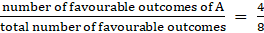= 0.5(ii) Probability of occurrence of event B = P(B)P(B) =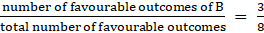= 0.375(iii) Probability of occurrence of event C = P(C)P(C) =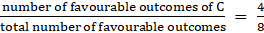= 0.5(iv) Probability of occurrence of event D = P(D)P(D) =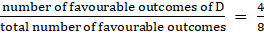= 0.5Question 9.A game of chance consists of spinning an arrow which comes to rest pointing at one of the numbers 1, 2, 3, 4, 5, 6, 7, 8, 9, 10, 11, 12 (see figure 16.1) and there are equally likely outcomes. What is the probability that it will point at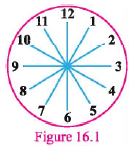(1) 7?(2) a number greater than 9?(3) an odd number?(4) an even number?(5) a number less than 5?Answer:Total numbers are from 1 to 12 i.e, 12 numbers.Now, let the event that the arrow will point at 7 be A.Event that arrow will point at a number greater than 5 be B.Event that arrow will point at an odd number be C.Event that arrow will point at an even number be D.Event that arrow will point at a number less than 5 be E.Number of favourable outcomes of event A = 1Favourable outcomes of event B i.e., a number greater than 5 are 6, 7, 8 ,9 10, 11, 12.Number of favourable outcomes of event B = 7Favourable outcomes of event C i.e., an odd number are 1,3,5,7,9,11.Number of favourable outcomes of event C = 6Favourable outcomes of event D i.e., an even number are 2,4,5,8,10,12.Number of favourable outcomes of event D = 6Favourable outcomes of event E i.e., a number less than 5 are 1,2 ,3,4.Number of favourable outcomes of event E = 4Total number of favourable outcomes = 12(i) Probability that the arrow will point at 7 = Probability of occurrence of event A = P(A)P(A) =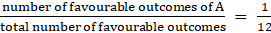= 0.0833(ii) Probability that arrow will point at a number greater than 5 = Probability of occurrence of event B = P(B)P(B) =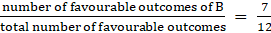= 0.58333(iii) Probability that arrow will point at an odd number = Probability of occurrence of event C = P(C)P(C) =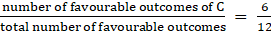= 0.5(iv) Probability that arrow will point at an even number = Probability of occurrence of event D = P(D)P(D) =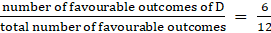= 0.5(v) Probability that arrow will point at a number less than 5 = Probability of occurrence of event E = P(E)P(E) =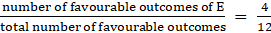= 0.3333Question 10.The sum of probability of event A and the probability of an event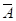(not A) is…..A. 0B. 1C. 0.5D. 0.4Answer:We know that P(A) + P(A’) = 1Question 11.The probability of the certain event is …..A. 0B. 0.5C. 0.7D. 1Answer:If an event is certain then it will surely occur.So, the probability of certain occurrence of event is always 1.Question 12.The probability of the impossible event is….A. 0B. 0.5C. 0.6D. 1Answer:If an event is impossible that means it will not occur in any case.So, the probability of impossible event is always 0.Question 13.The probability of an event is greater than or equal toA. 1B. 1.2C. 0.2D. 0Answer:The minimum probability of an event is 0 that is the event is not occurring.P(A)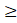0So, the probability of an event is greater than or equal to 0 .Question 14.The probability of an event is less than or equal to …….A. —1B. —0.1C. —0.5D. 1Answer:The maximum probability of an event is 1 that is the event is certainly occurring.P(A)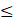1So, the probability of an event is less than or equal to 1 .Question 15.If P(A) = 0.35, then P(A’) = …..A. 0B. 0.35C. 0.65D. 1Answer:We know that P(A) + P(A’) = 1P(A) = 0.35P(A’) = 1-P(A) = 1- 0.35 = 0.65Question 16.If P(E) = 0.47, then P(E’) = ……..A. 0B. 0.20C. 0.50D. 0.53Answer:We know that P(E) + P(E’) = 1P(E) = 0.35P(E’) = 1-P(E) = 1- 0.47 = 0.53Question 17.The probability that you will get 101 marks in the paper which is in your hand is ……A. 1B. 0.5C. 0D. —0.5Answer:Assuming the paper in your hand is of total 100 marks and to get 101 out of 100 is an impossible event.Therefore, Probability of getting 101 marks out of 100 is 0.Question 18.The probability of the event "The Sun rises in West" is …..A. 1B. 0.5C. 0D. —0.5Answer:We know that The Sun rises in the East. It is a fact.So, it is an impossible event for the sun to rise in the west.Therefore, Probability of the event "The Sun rises in West" is 0Question 19.The sum of the probabilities of all the elementary events of an experiment is ….A. 0B. 0.2C. 1D. 0.8Answer:We know that the sum of probabilities of all the elementary events of an experiment is 1.

PDF FILE TO YOUR EMAIL IMMEDIATELY PURCHASE NOTES & PAPER SOLUTION. @ Rs. 50/- each (GST extra)

HINDI ENTIRE PAPER SOLUTION

MARATHI PAPER SOLUTION

SSC MATHS I PAPER SOLUTION

SSC MATHS II PAPER SOLUTION

SSC SCIENCE I PAPER SOLUTION

SSC SCIENCE II PAPER SOLUTION

SSC ENGLISH PAPER SOLUTION

SSC & HSC ENGLISH WRITING SKILL

HSC ACCOUNTS NOTES

HSC OCM NOTES

HSC ECONOMICS NOTES

HSC SECRETARIAL PRACTICE NOTES

# 2019 Board Paper Solution

HSC ENGLISH SET A 2019 21st February, 2019

HSC ENGLISH SET B 2019 21st February, 2019

HSC ENGLISH SET C 2019 21st February, 2019

HSC ENGLISH SET D 2019 21st February, 2019

SECRETARIAL PRACTICE (S.P) 2019 25th February, 2019

HSC XII PHYSICS 2019 25th February, 2019

CHEMISTRY XII HSC SOLUTION 27th, February, 2019

OCM PAPER SOLUTION 2019 27th, February, 2019

HSC MATHS PAPER SOLUTION COMMERCE, 2nd March, 2019

HSC MATHS PAPER SOLUTION SCIENCE 2nd, March, 2019

SSC ENGLISH STD 10 5TH MARCH, 2019.

HSC XII ACCOUNTS 2019 6th March, 2019

HSC XII BIOLOGY 2019 6TH March, 2019

HSC XII ECONOMICS 9Th March 2019

SSC Maths I March 2019 Solution 10th Standard11th, March, 2019

SSC MATHS II MARCH 2019 SOLUTION 10TH STD.13th March, 2019

SSC SCIENCE I MARCH 2019 SOLUTION 10TH STD. 15th March, 2019.

SSC SCIENCE II MARCH 2019 SOLUTION 10TH STD. 18th March, 2019.

SSC SOCIAL SCIENCE I MARCH 2019 SOLUTION20th March, 2019

SSC SOCIAL SCIENCE II MARCH 2019 SOLUTION, 22nd March, 2019

XII CBSE - BOARD - MARCH - 2019 ENGLISH - QP + SOLUTIONS, 2nd March, 2019

# HSCMaharashtraBoardPapers2020

(Std 12th English Medium)

HSC ECONOMICS MARCH 2020

HSC OCM MARCH 2020

HSC ACCOUNTS MARCH 2020

HSC S.P. MARCH 2020

HSC ENGLISH MARCH 2020

HSC HINDI MARCH 2020

HSC MARATHI MARCH 2020

HSC MATHS MARCH 2020

# SSCMaharashtraBoardPapers2020

(Std 10th English Medium)

English MARCH 2020

HindI MARCH 2020

Hindi (Composite) MARCH 2020

Marathi MARCH 2020

Mathematics (Paper 1) MARCH 2020

Mathematics (Paper 2) MARCH 2020

Sanskrit MARCH 2020

Sanskrit (Composite) MARCH 2020

Science (Paper 1) MARCH 2020

Science (Paper 2)

Geography Model Set 1 2020-2021

MUST REMEMBER THINGS on the day of Exam

Are you prepared? for English Grammar in Board Exam.

Paper Presentation In Board Exam

How to Score Good Marks in SSC Board Exams

Tips To Score More Than 90% Marks In 12th Board Exam

How to write English exams?

How to prepare for board exam when less time is left

How to memorise what you learn for board exam

No. 1 Simple Hack, you can try out, in preparing for Board Exam

How to Study for CBSE Class 10 Board Exams Subject Wise Tips?

JEE Main 2020 Registration Process – Exam Pattern & Important Dates

NEET UG 2020 Registration Process Exam Pattern & Important Dates

How can One Prepare for two Competitive Exams at the same time?

8 Proven Tips to Handle Anxiety before Exams!